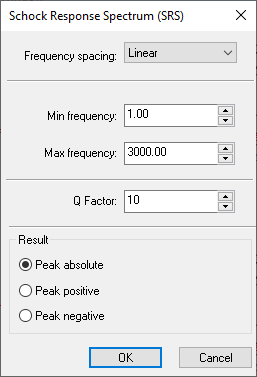Accessible through the “Signal tools/Shock Response Spectrum (SRS)” menu option.

Shock Response Spectrum (SRS) is a graphical representation of an arbitrary transient acceleration input, such as shock, in terms of how a Single Degree Of Freedom (SDOF) system (like a mass on a spring) responds to that input.

It actually shows the peak acceleration response of an infinite number of SDOFs, each of which have different natural frequencies. Peak acceleration response is represented on the Y-axis, and the natural frequency of any given SDOF oscillator is shown on the X-axis.

SRS plot in SIGVIEW can automatically use octave-based frequency spacing: you can choose between 1/24, 1/12, 1/6, 1/3 and linear frequency scaling. In addition, you can speed-up calculation by limiting the frequency range (min/max frequency values).

The level of damping is demonstrated by the "quality factor", Q which can also be thought of as transmissibility in sinusoidal vibration case. Relative damping of 5% results in a Q of 10.

Peak detection options determine how the algorithm detects the peak instantaneous acceleration.

After selecting the SRS option from the main menu, the following dialog will appear where you can set all calculation parameters:As a result, an SRS plot will appear in a new window.